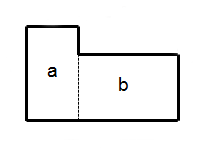Questions? 405-601-1300 M - F 10am to 5pm CST

# How Much Soil Do I Need?

Use This Soil Volume Calculator To calculate the amount of soil you need for raised beds, planters, pots, or vegetable gardens.

Soil Volume Calculator

Width: ft
Length: ft
Depth: in

Result: Yards

How to calculate the amount of soil needed for your garden in cubic feet or cubic yards

If your garden is a rectangle or square, doing some basic math to find the volume of soil, mulch, or compost you need isn't all that difficult. Using the graphic below as a reference, you can see we want to multiply in feet the length x width x height ÷ 27 to get the volume in cubic yards of soil that you need.To get the amount of cubic feet of soil instead of cubic yards, you would take you measurements in feet & multiply the length x width x depth = how many cubic feet of product you need to fill the volume of this garden or raised bed.

If you have an odd shaped yard or flower bed, you can break the area up into different quadrants, and once you have done the math on each quadrant you can add all of the quadrants together. Make sure you measurements are in feet when finding the area of each quadrant.

Area a: L x W x D ÷ 27 = Cubic Yards

Area b: L x W x D ÷ 27 = Cubic Yards

Now to get the total volume of the area in cubic yards you would add a & b

Area a + Area b = Total volume in cubic yardsIf you are wanting to calculate in cubic feet instead of cubic yards for the same area, you would do the following math. Start with measuring the perimeters in feet..

Area a: L x W x D = Cubic Feet

Area b: L x W x D = Cubic Feet

Now to get the total volume of the area you would add a & b

Area a + Area b = Total volume in cubic feet

When it comes to more complex shaped yards, you can use our soil volume calculator above to calculate the volume in cubic yards of how much material you need to fill pretty much any given shape. If your area is an irregular shape, split it up into different quadrants, and enter them into the calculator, then add all of the sums together to get the total amount of volume in yards needed of mulch, soil, compost, etc.. needed for your project. Whether your area is a square, a rectangle, triangles, or circles, you can use our calculator to figure out how many yards of material you need to fill your garden or flower beds with soil.

Our volume calculator can be used to calculate the amount of gravel needed for a driveway, the amount of mulch needed for your flower beds, or the amount of compost needed for your farm or garden. It can be used to calculate the amount of yards needed for pretty much anything you buy in yards. The applications really are endless.

Spend more time in the garden, and less time doing math. We hope our free soil volume calculator will help you achieve your goals, and make your life a little easier so you can get back to what you love to do, gardening.